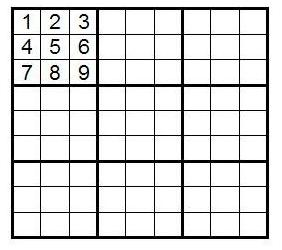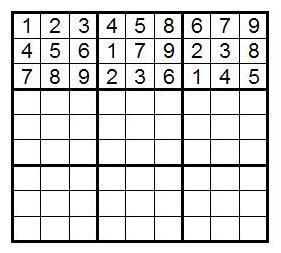### Contents

Home

1. Introduction to Sudoku

2. Solving Strategy

3. Counting Solutions

4. Solution Symmetries

5. The 4×4 Case

6. Some More Interesting Facts

7. References

# The Math Behind Sudoku

## Counting Solutions

An interesting question to ask is how many ways can a 9 by 9 Sudoku grid be filled so that it satisfies the One Rule? In other words, how many distinct Sudoku solutions are there? We describe the method used to calculate this number by Bertram Felgenhauer and Frazer Jarvis in early 2006.

To keep our language standard, we call the three rows of blocks the bands of the grid and the three columns of blocks the stacks. A cell in the ith row and jth column is said to be in (i,j) position.

We call the number of distinct Sudoku grids N. First we label the blocks of the grid as follows:How many ways are there to fill B1 in a valid way? Since there are 9 symbols that can fill up B1, one in each cell, there are 9 options for the first cell. For each of these 9 options there are 8 options remaining for second cell. For each of these 8 options, there are 7 left for the third cell. We are essentially computing the number of permutations of 9 symbols: how many ways we can arrange 9 symbols into 9 places, or how many ways we can order 9 things. There are 9! ways of filling in B1. Starting with a valid B1 block, we can obtain any other valid B1 block by re-labeling, or permuting, the numbers. So the first simplifying assumption we can make is that B1 is filled in with the numbers 1, 2, 3, ..., 9 in order, as shown below.Now we can calculate how many valid grid completions this particular B1 has: call it N1. The total number of valid Sudoku grids will be N1×9!, so N1=N/9!.

We consider the ways to fill in the first rows in B2 and B3. Since 1, 2, and 3 occur in the first row in B1, these numbers cannot occur in the rest of the row. So only the numbers 4, 5, 6, 7, 8, and 9 from the second and third rows of B1 can be used in the first row in B2 and B3.

Exercise: List all the possible ways of filling in the first rows in B2 and B3, up to reordering of the digits in each block. Hint: There are ten ways of doing this so that swapping B2 and B3 in these ten ways give you ten more ways, for a total of twenty.

We call two of these possibilities the pure top rows: when the numbers {4,5,6} as in the second row of B1 are kept together in B2 and the numbers {7,8,9} as in the third row of B1 are kept together in B3, and the swapped version of this. The rest of the possibilites are mixed top rows, since they mix up the sets {4,5,6} and {7,8,9} when using them to fill in the first rows of B2 and B3.

Given these twenty possibilities for the first row of the grid (up to reordering of the digits in each block), we can figure out how to complete the first band.

Exercise: Think about how you can complete the first band starting with the pure top row 1,2,3;{4,5,6};{7,8,9}. (Note: We are writing a,b,c to denote an ordered triple of numbers and {a,b,c} to denote them in any order.) How many total possibilities are there, counting different number orderings within each row in B2 and B3? Is this number the same as for the other pure top row, 1,2,3;{7,8,9};{4,5,6}?

Remember, we want to keep B1 fixed because we've already accounted for the number of grids that can be obtained by relabeling the nine digits in it.

It turns out there are (3!)6 ways to complete the first band staring with the pure top row 1,2,3;{4,5,6};{7,8,9}. This is because we are forced to put {7,8,9};{1,2,3} in the second row in B2 and B3 and {1,2,3};{4,5,6} in the third row, and then we may reorder the three digits in each of the six rows of B2 and B3 to get all the configurations. The answer is the same for the other pure top row, since in this case all we've done is swapped B2 with B3.

The cases of the mixed top rows are more complicated. Let's consider the top row 1,2,3;{4,6,8};{5,7,9}. This can be completed to the first band as in the following picture, where a, b, and c are the numbers 1, 2, 3 in any order.Once a is picked, b and c are the remaining two numbers in any order, since they are in the same row. Since there are three choices for a, and the three digits in each of the six sets in B2 and B3 can be permuted to result in various valid first bands, the total number of configurations in this case is 3×(3!)6. You can similarly work out each of the remaining seventeen cases of first rows to obtain the same number.

Now we have the number of possible first bands given the standard B1: it is 2×(3!)6+18×3×(3!)6=2612736, where the first part of the sum is the number of first band completions from the pure top rows and the second is the number of first band completions from the mixed top rows.

Instead of calculating how many full grid completions each of these 2612736 possibilities has, Felgenhauer and Jarvis next determined which first bands share the same number of full grid completions. Such an analysis reduces the number of first bands that need to be considered when counting.

Here are some operations which leave the number of grid completions of the first band unchanged: relabeling the numbers, permuting any of the blocks in the first band, permuting the columns within any block, and permuting the three rows of the band. When any of these changes B1, we can relabel the digits to recover its standard form.

Permuting B1, B2, and B3 preserves the number of grid completions because if we start with any valid Sudoku grid, the only way to keep it valid would be to permute B4, B5, B6 and B7, B8, B9 correspondingly so that the stacks remain the same. In other words, every valid grid completion for the first band gives exactly one valid grid completion for the first band being any permutation of B1, B2, B3.

Exercise: Convince yourself that when you swap B2 with B3 in the following Sudoko grid, the only way to keep the grid valid is to swap B5 with B6, and B7 with B8. This keeps the stacks the same, although their locations have changed.Exercise: If you have a valid Sudoku grid and you permute the columns in any of B1, B2, and B3, what would you need to do to the columns of the rest of the Sudoku grid in order to keep it valid? For example, if you swap columns 1 and 2 of B2 in the above grid, how would you fix the resulting grid so that it satisfies the One Rule?

The last exercise tells us that each grid completion of a first band gives a unique grid completion of the first band with columns permuted within the blocks.

Such considerations allow us to reduce the number of specific first bands we need to consider when counting. Following Felgenhauer and Jarvis, we permute the columns of B2 and B3 so that the top row entries of each are in increasing order, and then swap B2 and B3 if necessary to make the first entry of B2 smaller than that of B3. This is called lexicographical reduction. Since there are 6 permutations of the columns in each of the two blocks and two ways to permute the blocks, lexicographical reduction tells us that, given a first band, there are 62×2=72 other first bands with the same number of grid completions. So now we only need to consider 2612736/72=36288 first bands.

For each of these possibilities, we consider permutations of all three top blocks: there are 6 of them. For each of these, there are 63 permutations of the columns within each block. After performing these operations, we relabel to get B1 back into standard form. We can similarly permute the top three rows of the band, and relabel to get B1 back into standard form. These operations preserve the number of grid completions of a first band. Felgenhauer and Jarvis used a computer program to determine that these operations reduce the number of first bands to be considered from 36288 to just 416.

Exercise: Consider the given first band. Perform various operations on it that preserve its number of grid completions. You can start with the following: Exchange the first and second row. Relabel so that B1 is in standard form. Lexicographically reduce this grid.The main point is that the band you started with and the different band you ended with have the same number of completions to a full Sudoku grid. So instead of calculating the number of grid completions for each of these bands, we can count it for just one of them.

There are more steps that reduce the number of grids to be considered. If we have a pair of digits {a,b} in one column with a in the ith row and b in the jth row, and the same pair in a different column with b in the ith row and a in the jth row, then swapping the places of a and b in each pair will result in a band that has the same number of grid completions as the original. This is because each pair lies in the same column, and swapping both at once keeps the One Rule satisfied for the rows involved as well. As an example, look at the numbers 8 and 9 in the sixth and ninth columns of the above example. Considering all possible cases of this left Felgenhauer and Jarvis with 174 out of the 416 first bands with which to proceed.

They also considered other configurations of the same set of digits lying in two different columns or rows, which can be permuted within their columns or rows leaving the number of grid completions invariant. This reduced the number of first bands to consider to 71, and searching through each of these 71 cases let them know that there are actually only 44 first bands whose number of grid completions need to be found. Each of these 44 bands has the same number of completions to a full grid.

Let C stand for one of these 44 bands. Then the number of ways that C can be completed to a full Sudoku grid can be calculated: call it nc. We also need the number mC of first bands that share this number nC of grid completions. Then the total number of Sudoku grids will just be N=ΣCmCnC, or the sum of mCnC over all of the 44 bands.

Felgenhauer and Jarvis wrote a computer program to carry out the final calculations. They computed the number N1 of valid completions with B1 in standard form, starting with the 44 bands. Then they multiplied this number by 9! to get the answer. They discovered that the number of possible 9 by 9 Sudoku grids is N=6670903752021072936960 which is approximately 6.671×1021.